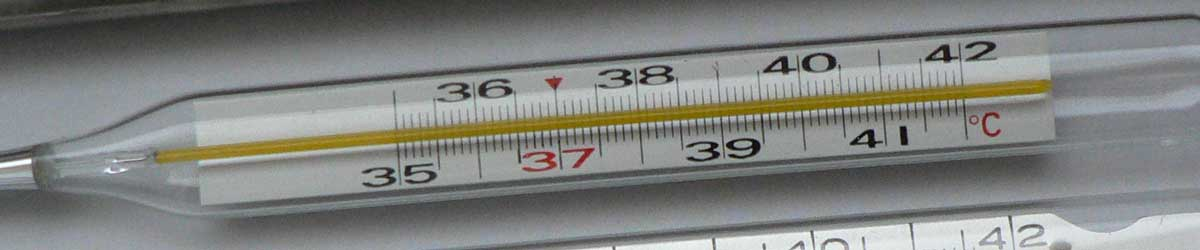Convert Fahrenheit to Kelvins

Enter the temperature in Fahrenheit below to get the value converted to kelvins.

Results in Kelvins:1 °F = 255.927778 K

How to Convert Fahrenheit to KelvinsConvert degrees Fahrenheit to kelvins with this simple formula:

kelvins = ( ( [°F] - 32 ) × 5 / 9 ) + 273.15

Insert the [°F] temperature measurement in the formula and then solve to find the result.

For example, let's convert 50 °F to kelvins:

50 °F = ( ( ( 50 - 32 ) × 5 / 9 ) + 273.15 ) = 283.15 K

Fahrenheit and kelvins are both units used to measure temperature. Keep reading to learn more about each unit of measure.

Fahrenheit

The Fahrenheit scale is a temperature scale that defines the melting point of water as 32 degrees and the boiling point of water at 212 degrees. There are 180 intervals between 32 °F and 212 °F, each corresponding to one degree.

The degree Fahrenheit is a US customary and imperial unit of temperature. Fahrenheit can be abbreviated as F, for example 1 degree Fahrenheit can be written as 1 °F.

Important Temperatures In Degrees Fahrenheit

TemperatureDegrees Fahrenheit
Absolute Zero-459.67 °F
Freezing Point of Water32 °F
Triple Point of Water32.018 °F
Boiling Point of Water212 °F

Kelvins

The Kelvin scale is an absolute temperature scale that uses absolute zero as the starting point. The kelvin is defined in terms of the Boltzmann constant, which is a measure of the energy of motion corresponding to one kelvin. 0 kelvins are equal to −273.15 degrees Celsius, which is the point of absolute zero.

The kelvin is the SI base unit for temperature in the metric system. Kelvins can be abbreviated as K, for example 1 kelvin can be written as 1 K.

The kelvin scale is different from other temperature scales in that it is not expressed in degrees, values are expressed in kelvins. When using the Kelvin scale in writing, the "k" is capitalized, however when using the kelvin as a unit the "k" should be lower-case, even though it is named for a person.

Important Temperatures In Kelvins

TemperatureKelvins
Absolute Zero0 K
Freezing Point of Water273.15 K
Triple Point of Water273.16 K
Boiling Point of Water373.15 K

Fahrenheit Measurements and Equivalent Kelvin Conversions

Common Fahrenheit values converted to the equivalent kelvin value
Fahrenheit Kelvins
-50 °F 227.59 K
-40 °F 233.15 K
-30 °F 238.71 K
-20 °F 244.26 K
-10 °F 249.82 K
0 °F 255.37 K
10 °F 260.93 K
20 °F 266.48 K
30 °F 272.04 K
40 °F 277.59 K
50 °F 283.15 K
60 °F 288.71 K
70 °F 294.26 K
80 °F 299.82 K
90 °F 305.37 K
100 °F 310.93 K
110 °F 316.48 K
120 °F 322.04 K
130 °F 327.59 K
140 °F 333.15 K
150 °F 338.71 K
160 °F 344.26 K
170 °F 349.82 K
180 °F 355.37 K
190 °F 360.93 K
200 °F 366.48 K
300 °F 422.04 K
400 °F 477.59 K
500 °F 533.15 K
600 °F 588.71 K
700 °F 644.26 K
800 °F 699.82 K
900 °F 755.37 K
1000 °F 810.93 K

More Fahrenheit Temperature Conversions

Convert to Celsius
1 °F is equal to -17.222222 °C
Convert to Rankine
1 °F is equal to 460.67 °R
Convert to Delisle
1 °F is equal to 175.833333 °De
Convert to Newton
1 °F is equal to -5.683333 °N